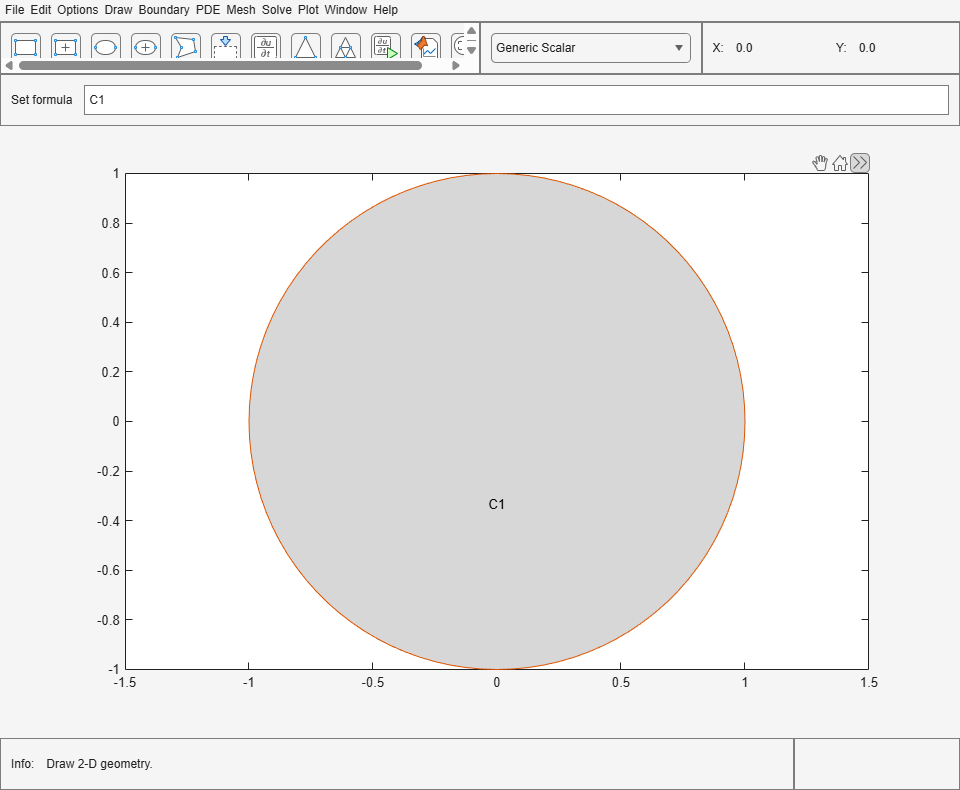# pdecirc

Draw circle in PDE Modeler app

## Syntax

``pdecirc(xc,yc,R)``
``pdecirc(xc,yc,R,label)``

## Description

example

````pdecirc(xc,yc,R)` draws a circle with the center at (`xc`,`yc`) and the radius `R`. The `pdecirc` command opens the PDE Modeler app with the specified circle already drawn in it. If the app is already open, `pdecirc` adds the specified circle to the app window without deleting any existing shapes.`pdecirc` updates the state of the geometry description matrix inside the PDE Modeler app to include the circle. You can export the geometry description matrix from the PDE Modeler app to the MATLAB® Workspace by selecting DrawExport Geometry Description, Set Formula, Labels.... For details on the format of the geometry description matrix, see `decsg`.```

example

````pdecirc(xc,yc,R,label)` assigns a name to the circle. Otherwise, `pdecirc` uses a default name, such as `C1`, `C2`, and so on.```

## Examples

collapse all

Open the PDE Modeler app window containing a circle with the center at (0,0) and the radius 1.

`pdecirc(0,0,1)`

Call the `pdecirc` command again to draw a circle with the center at (0,0.25) and the radius 0.5. The `pdecirc` command adds the second circle to the app window without deleting the first.

`pdecirc(0,0.25,0.5)`Open the PDE Modeler app window containing a circle with the center at (0,0) and the radius 1. Assign the name `circle1` to this circle.

`pdecirc(0,0,1,'circle1')`## Input Arguments

collapse all

x-coordinate of the center of the circle, specified as a real number.

Data Types: `double`

y-coordinate of the center of the circle, specified as a real number.

Data Types: `double`

Radius of the circle, specified as a positive number.

Data Types: `double`

Name of the circle, specified as a character vector or string scalar.

Data Types: `char` | `string`

## Tips

`pdecirc` opens the PDE Modeler app and draws a circle. If, instead, you want to draw circles in a MATLAB figure window, choose one of these approaches:

• Use the `plot` command, for example:

```t = linspace(0,2*pi); plot(cos(t),sin(t))```
• Use the `rectangle` function with the `Curvature` name-value pair set to ```[1 1]```.

• Use the Image Processing Toolbox™ `viscircles` (Image Processing Toolbox) function.